# RD Sharma Solutions for Class 8 Math Chapter 8 - Division of Algebraic Expressions (Part-2) Notes | Study RD Sharma Solutions for Class 8 Mathematics - Class 8

## Class 8: RD Sharma Solutions for Class 8 Math Chapter 8 - Division of Algebraic Expressions (Part-2) Notes | Study RD Sharma Solutions for Class 8 Mathematics - Class 8

The document RD Sharma Solutions for Class 8 Math Chapter 8 - Division of Algebraic Expressions (Part-2) Notes | Study RD Sharma Solutions for Class 8 Mathematics - Class 8 is a part of the Class 8 Course RD Sharma Solutions for Class 8 Mathematics.
All you need of Class 8 at this link: Class 8

#### Question 1: Divide x + 2x2 + 3x4 − x5 by 2x.Answer 1: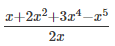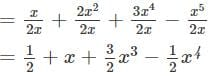Question 2: Divide y43y3+1/2 y2 by 3yy4-3y3+12y2 by 3y.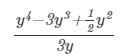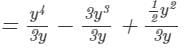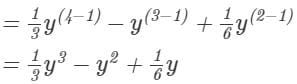#### Question 3: Divide −4a3 + 4a2 + a by 2a.Answer 3: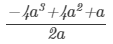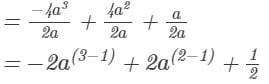=2a2+2a+1/2
Question 4: Divide x6+2x4+4x3+2x2 by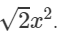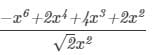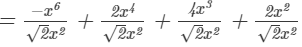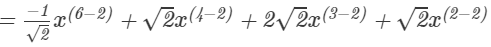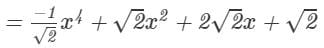#### Question 5: Divide 5z3 − 6z2 + 7z by 2z.Answer 5: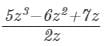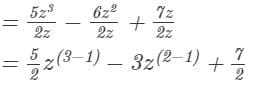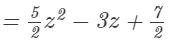Question 6: Divide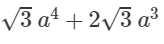+3a26a by 3a3 a4+23 a3+3a2-6a by 3a.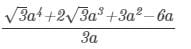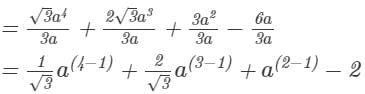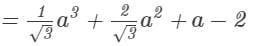#### Question 7: Divide 5x3 − 15x2 + 25x by 5x.Answer 7: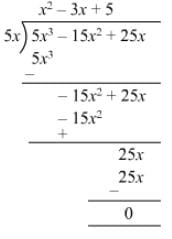Question 8: Divide 4z3 + 6z2z by −1/2 12z.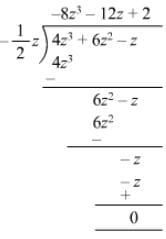#### Question 9: Divide 9x2y − 6xy + 12xy2 by −3/2 32xy.Answer 9: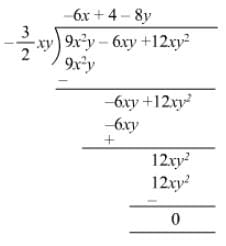Question 10: Divide 3x3y2 + 2x2y + 15xy by 3xy.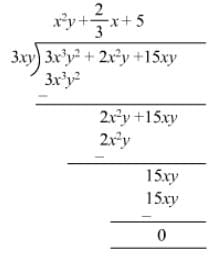Question 11: Divide x2 + 7x + 12 by x + 4.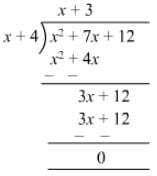####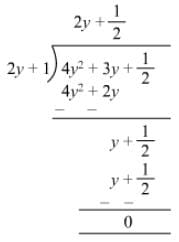Question 13: Divide 3x3 + 4x2 + 5x + 18 by x + 2.Answer 13: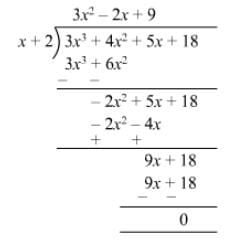Question 14: Divide 14x2 − 53x + 45 by 7x − 9.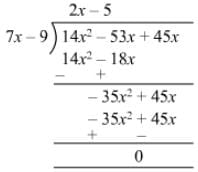Question 15: Divide −21 + 71x − 31x2 − 24x3 by 3 − 8x.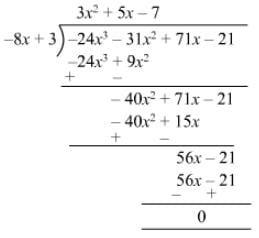Question 16: Divide 3y4 − 3y3 − 4y2 − 4y by y2 − 2y.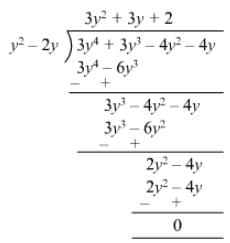Question 17: Divide 2y5 + 10y4 + 6y3 + y2 + 5y + 3 by 2y3 + 1.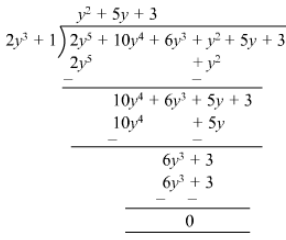Question 18: Divide x4 − 2x3 + 2x2 + x + 4 by x2 + x + 1.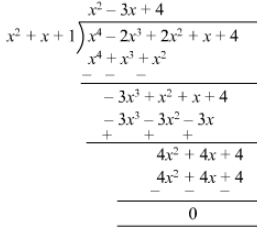Question 19: Divide m3 − 14m2 + 37m − 26 by m2 − 12m +13.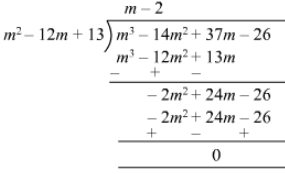Question 20: Divide x4 + x2 + 1 by x2 + x + 1.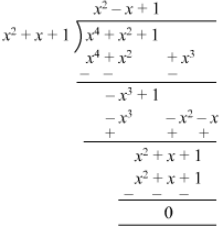Question 21: Divide x5 + x4 + x3 + x2 + x + 1 by x3 + 1.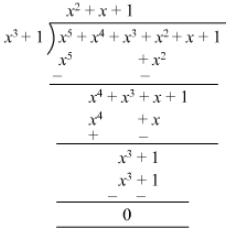Question 22: Divide 14x3 − 5x2 + 9x − 1 by 2x − 1 and find the quotient and remainder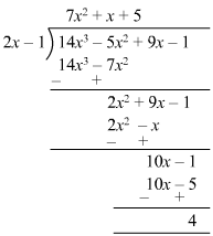Quotient = 7x2 + x + 5Remainder = 4

#### Question 23: Divide 6x3 − x2 − 10x − 3 by 2x − 3 and find the quotient and remainder.Answer 23: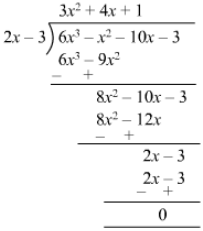Quotient = 3x2+ 4x + 1 Remainder = 0Question 24: Divide 6x3 + 11x2 − 39x − 65 by 3x2 + 13x + 13 and find the quotient and remainder.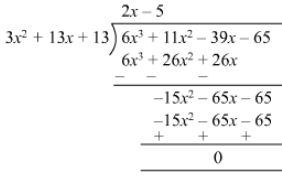Quotient = 2x5Remainder =

#### Question 25: Divide 30x4 + 11x3 − 82x2 − 12x + 48 by 3x2 + 2x − 4 and find the quotient and remainder.Answer 25: Quotient =10x2−3x−12 Remainder= 0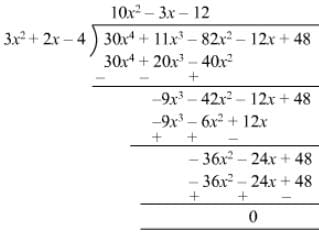Question 26: Divide 9x4 − 4x2 + 4 by 3x2 − 4x + 2 and find the quotient and remainder.Answer 26: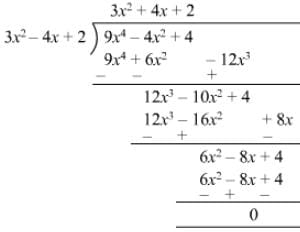Quotient = 3x2 + 4x + 2 and remainder = 0.
Question 27: Verify the division algorithm i.e. Dividend = Divisor × Quotient + Remainder, in each of the following. Also, write the quotient and remainder.

 Dividend Divisor 14x2 + 13x − 15 7x − 4 15z3 − 20z2 + 13z − 12 3z − 6 6y5 − 28y3 + 3y2 + 30y − 9 2y2 − 6 34x − 22x3 − 12x4 − 10x2 − 75 3x + 7 15y4 − 16y3 + 9y2 − 10/3 103y + 6 3y − 2 4y3 + 8y + 8y2 + 7 2y2 − y + 1 6y5 + 4y4 + 4y3 + 7y2 + 27y + 6 2y3 + 1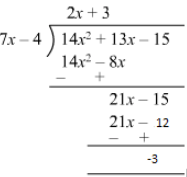Quotient = 2x + 3
Remainder = -3
Divisor = 7x - 4
Divisor ×× Quotient + Remainder = (7x - 4) (2x + 3) - 3

= 14x+ 21- 8- 12 - 3
= 14x2 + 13x - 15
= Dividend

Thus,
Divisor ×× Quotient + Remainder = Dividend
Hence verified.

(ii)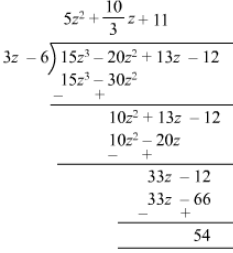Quotient = 5z2+10/3 z+11Remainder = 54Divisor = 3z6Divisor × Quotient +Remainder = (3z6)( 5z2+10/3 z+11)+54= 15z3+10z2+33z30z220z66+54

= 15z320z2+13z12

= Dividend
Thus,Divisor × Quotient + Remainder = DividendHence verified.
(iii)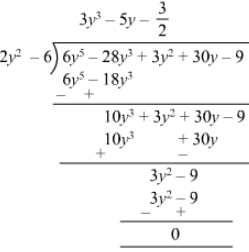Quotient = 3y35y+323y3-5y+32
Remainder = 0
Divisor = 2y2 - 6
Divisor ×× Quotient + Remainder =
(2y26) (3y35y+32)+0=6y510y3+3y218y3+30y9=6y528 y3+3y2+30y9 = Dividend

Thus, Divisor ×× Quotient + Remainder = Dividend
Hence verified.

(iv)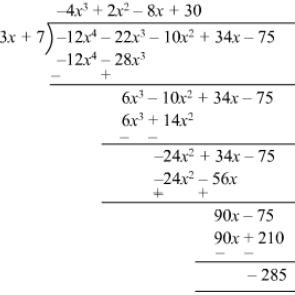Quotient  = - 4x3 + 2x2 - 8x + 30
Remainder  = - 285
Divisor  = 3x + 7
Divisor ×× Quotient + Remainder =  (3x + 7) (- 4x3 + 2x2 - 8x + 30) - 285

- 12x4 + 6x3 - 24x2 + 90- 28x3 + 14x2 - 56x + 210 - 285
- 12x 4 - 22x3 - 10x2 + 34x - 75
=  Dividend

Thus,
Divisor ×× Quotient + Remainder = Dividend
Hence verified.

(v)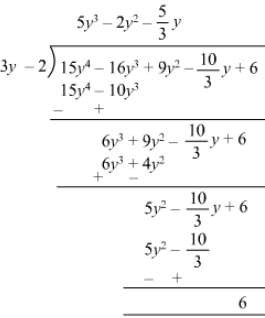Quotient =  5y32y2+5/3 y5y3-2y2+53y
Remainder =  6
Divisor = 3y - 2
Divisor ×× Quotient  + Remainder = (3y - 2) (5y3 - 2y2 + 5/3 y53y) + 6

15y46y3+5y210y3+4y210/3 y+615y4-6y3+5y2-10y3+4y2-103y+6
15y416y3+9y210/3 y+615y4-16y3+9y2-103y+6
=  Dividend

Thus,
Divisor ×× Quotient + Remainder = Dividend
Hence verified.

(vi)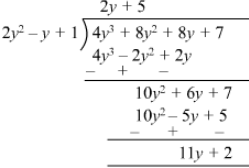Quotient =  2y + 5
Remainder =  11y + 2
Divisor =  2y2 - y + 1
Divisor ×× Quotient + Remainder =  (2y2 - y + 1) (2y + 5) + 11y + 2

=  4y3 +10y2 - 2y2 - 5y + 2y + 5 + 11y + 2
=  4y3 + 8y2 + 8y + 7
=  Dividend
Thus,
Divisor ×× Quotient + Remainder  = Dividend
Hence verified.

(vii)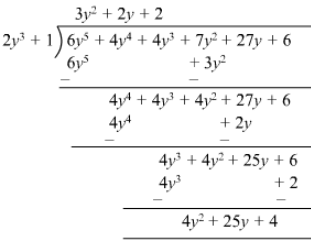Quotient = 3y2 + 2y + 2
Remainder = 4y2 + 25y + 4
Divisor = 2y3 + 1
Divisor ×× Quotient + Remainder = (2y3 + 1) (3y2 2y + 2) + 4y2 + 25y + 4

6y5 + 4y4 + 4y3 + 3y2 + 2y + 4y2 + 25y + 4
6y5 + 4y4 + 4y3 + 7y2 + 27y + 6
= Dividend

Thus,
Divisor ×× Quotient + Remainder = Dividend
Hence verified.

#### Question 28: Divide 15y4 + 16y3 + 10/3 103y − 9y2 − 6 by 3y − 2. Write down the coefficients of the terms in the quotient.Answer 28: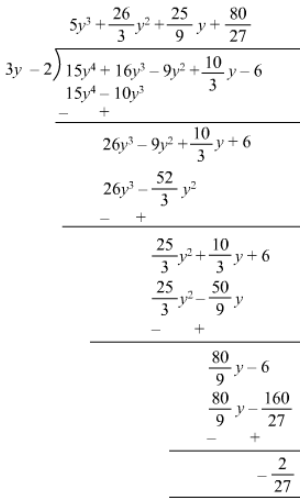Quotient = 5y3 + (26/3)y2 + (25/9)y + (80/27)
Remainder = (- 2/27)
Coefficient of y3 = 5
Coefficient of y2 = (26/3)
Coefficient of y = (25/9)
Constant = (80/27)

#### Question 29: Using division of polynomials, state whether(i) x + 6 is a factor of  x2 − x − 42(ii) 4x − 1 is a factor of 4x2 − 13x − 12(iii) 2y − 5 is a factor of 4y4 − 10y3 − 10y2 + 30y − 15(iv) 3y2 + 5 is a factor of 6y5 + 15y4 + 16y3 + 4y2 + 10y − 35(v) z2 + 3 is a factor of z5 − 9z(vi) 2x2 − x + 3 is a factor of 6x5 − x4 + 4x3 − 5x2 − x − 15Answer 29: (i)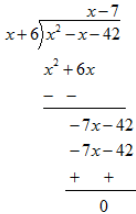Remainder is zero. Hence (x+6) is a factor of x2 -x-42
(ii)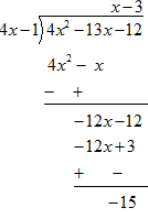As the remainder is non zero . Hence ( 4x-1) is not a factor of 4x2 -13x-12
(iii)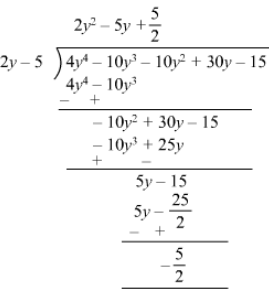The remainder is non zero,
2y - 5 is not a factor of 4y410y310y2+30y154y4-10y3-10y2+30y-15.

(iv)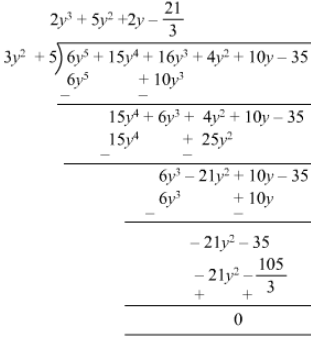Remainder is zero.  Therefore, 3y2 + 5 is a factor of 6y5+15y4+16y3+4y2+10y356y5+15y4+16y3+4y2+10y-35.
(v)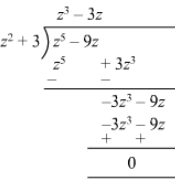Remainder is zero; therefore, z2 + 3 is a factor of z5 9zz5 -9z.
(vi)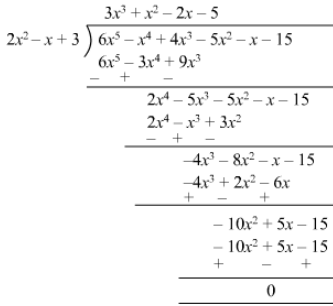Remainder is zero ; therefore, 2x2x+32x2-x+3 is a factor of 6x5x4 +4x35x2x156x5-x4 +4x3-5x2-x-15.
Question 30: Find the value of a, if x + 2 is a factor of 4x4 + 2x3 − 3x2 + 8x + 5a.

Answer 30:  We have to find the value of a if (x+2) is a factor of (4x4+2x3−3x2+8x+5a).Substituting x=2 in 4x4+2x33x2+8x+5a, we get:4(2)4+2(2)33(2)2+8(2)+5a=0or, 64161216+5a=0or, 5a=20or, a=4 If (x+2) is a factor of (4x4+2x33x2+8x+5a), a=4.Question 31: What must be added to x4 + 2x3 − 2x2 + x − 1 , so that the resulting polynomial is exactly divisible by x2 + 2x − 3?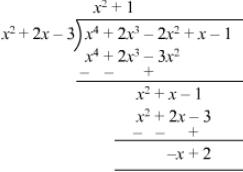Thus, (x - 2) should be added to (x4+2x32x2+x1x4+2x3-2x2+x-1) to make the resulting polynomial exactly divisible by (x2+2x3x2+2x-3).

The document RD Sharma Solutions for Class 8 Math Chapter 8 - Division of Algebraic Expressions (Part-2) Notes | Study RD Sharma Solutions for Class 8 Mathematics - Class 8 is a part of the Class 8 Course RD Sharma Solutions for Class 8 Mathematics.
All you need of Class 8 at this link: Class 8Use Code STAYHOME200 and get INR 200 additional OFF

## RD Sharma Solutions for Class 8 Mathematics

88 docs

Track your progress, build streaks, highlight & save important lessons and more!

,

,

,

,

,

,

,

,

,

,

,

,

,

,

,

,

,

,

,

,

,

;